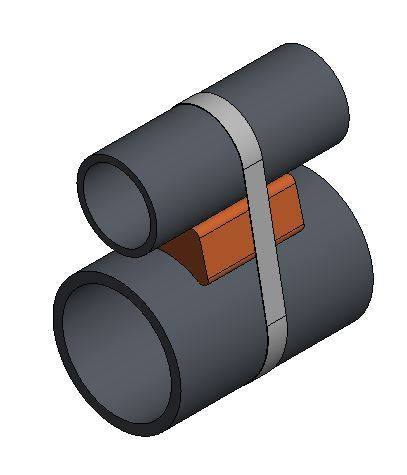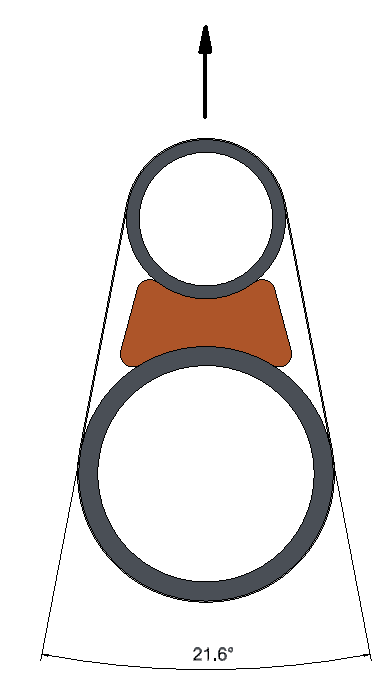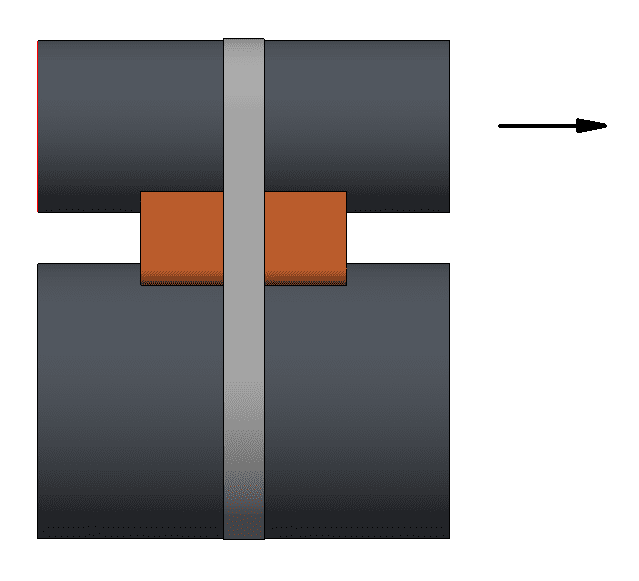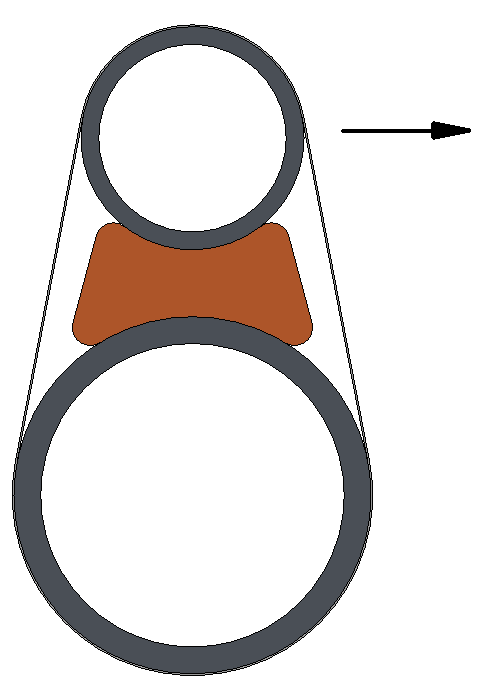Homework Statement:
Two pipes are strapped together using a single carbon steel band with a spacer in between. Assuming the larger diameter pipe is fixed, I am trying to calculate the following three scenarios:
The maximum pull off load away from the larger diameter
Relevant Equations:
See text body
Imagine the following scenario. A pipe is strapped to a larger "host" pipe using a steel strap and a spacer block as shown in the image below. If anyone could have a look at my calculations to confirm they are correct that would be brilliant!Variables
Assuming the following general values I would like to calculate the three maximum loads mentioned in the title:
Diameter of large pipe (DL) = 200mm
Diameter of small pipe (DS) = 125mm
Pipe separation C-C (Sep) = 200mm
Coefficient of Friction (CoF) = 0.2
Factor of Safety (FoS) = 1.5
Factor for Capstan effect (Cf) = 1.3 (loss in tension due to friction of band on pipes)
Strap width (Sw) = 30mm
Strap thickness (St) = 1mm
Strap Tensile Strength (σ) = 370MPa
Strap Tension (T) = 400kg
Strap Angle (θ) = 26.1° / 0.38rad

Pull off Capacity
The pull off capacity is defined by the yield strength of the strap. Rearranging the standard Stress = Force/Area equation gives a yield force for our strap material of:
Yield Force (Fy) = 370 * (30 * 1) = 11.1kN
Since the strap is at an angle, only a component of it is resisting the pull of the smaller pipe. The vertical component of this force is calculated using trigonometry. The force will act over two cross sections of band. Adding in a material utilisation factor gives the pull off force to result in strap yield is:
Fy * 2 * cos(θ / 2) * 0.67 = 14.6kN or 1489kgAxial Capacity
The strap is pressing the smaller diameter pipe into the spacer, and then the "fixed" larger diameter pipe. Again since the strap is at an angle only a component of the tension is clamping the small pipe down:
Vertical component of strap tension (Tv) = 2 * T * cos(θ/2), which in this case equals 2 * 400 * cos(0.19) = 785.6kg
Max Axial Load = (Tv * CoF) / (FoS * Cf) = (785.6 * 0.2) / (1.5 * 1.3) = 80.6kgThe radial capacity is a bit more complicated to calculate.
Applying a load (L) as shown in the image below creates a moment force (MF) = L * Sep
The force required at the interface between the spacer and the larger diameter pipe to resist the moment (FRM) is: MF / (DL / 2)
Accounting for the friction, the clamp force (CF) required is: FRM / CoF
The strap tension required TR is therefore = cos(θ / 2) * CF / 2
Again accounting for FoS and Capstan effect, the tension required to resist load L = TR * FoS * Cf
Plugging in our values we can combine the equations to give:
T = (L * FoS * Cf * Sep * cos(θ / 2) ) / (CoF * DL)
400 = (L * 1.5 * 1.3 * 0.2 * cos(0.19)) / (0.2 * 0.2)
L = 41.8kgLast edited by a moderator: